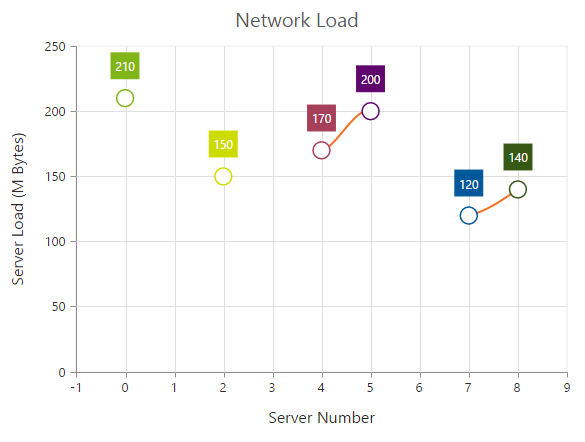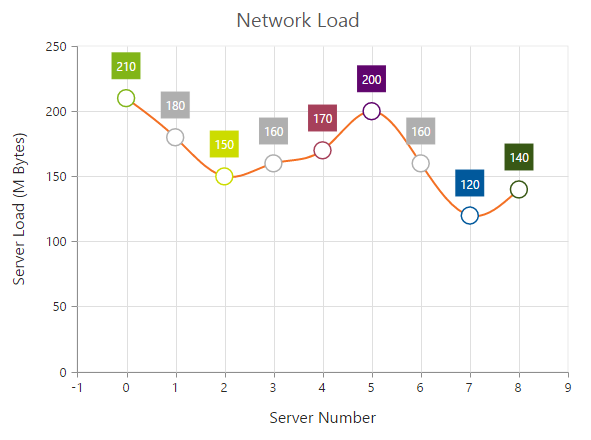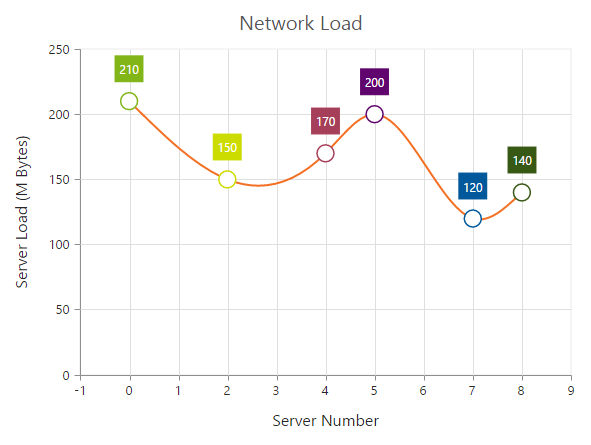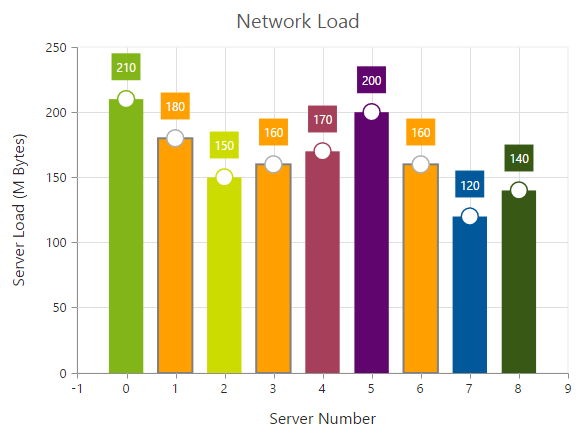# Empty Points

8 Jun 20173 minutes to read

The Data points that uses the null or undefined as value are considered as empty points. Empty data points are ignored and not plotted in the Chart. When the data is provided by using the `points` property, you can set the isEmpty to true to specify that the particular point is an empty point.

• HTML
• ``````<ej-chart id="chartcontainer">
<e-seriescollection>
<e-series type="spline">
<e-points>
<e-point x="0" [y]="210"></e-point>
<e-point x="1" [y]="null"></e-point>
<e-point x="2" [y]="150"></e-point>
<e-point x="3" [y]="180" [isEmpty]="true"></e-point>
<e-point x="4" [y]="170"></e-point>
<e-point x="5" [y]="200"></e-point>
<e-point x="6" [y]="140" [isEmpty]="true"></e-point>
<e-point x="7" [y]="120"></e-point>
</e-points>
</e-series>
</e-seriescollection>
</ej-chart>``````## EmptyPointSettings

You can customize the empty points visibility and change its `displayMode` (gap, zero and average) using `emptyPointSettings` option.

• HTML
• ``````<ej-chart id="chartcontainer">
<e-seriescollection>
<e-series [emptyPointSettings.visible]="true" emptyPointSettings.displayMode="average">
</e-series>
</e-seriescollection>
</ej-chart>``````If the `visible` property of `emptyPointSettings` is false, then the empty points has been dropped and chart will be rendered without empty points.## Customizing Styles

Empty points color and border can be customized using `style` property of `emptyPointSettings`.

• TS
• ``````this.emptyStyle = {
color: "#ffa000",
border: {
color: "gray",
width: 2
}
};``````
• HTML
• ``````<ej-chart id="chartcontainer">
<e-seriescollection>
<e-series [emptyPointSettings.visible]="true" [emptyPointSettings.style]="emptyStyle">
</e-series>
</e-seriescollection>
</ej-chart>``````